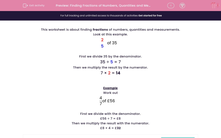# Finding Fractions of Numbers, Quantities and Measurements

In this worksheet, students find fractions of numbers, quantities and measurements.This content is premium and exclusive to EdPlace subscribers.Key stage:  KS 2

Curriculum topic:   Maths and Numerical Reasoning

Curriculum subtopic:   Mixed Problems

Difficulty level:#### Worksheet Overview

This worksheet is about finding fractions of numbers, quantities and measurements.

Look at this example.

 2 5
of 35

First we divide 35 by the denominator.

35 ÷ 5 = 7

Then we multiply the result by the numerator.

7 × = 14

Example

Work out

 4 7
of £56

First we divide with the denominator.

£56 ÷ 7 = £8

Then we multiply the result with the numerator.

£8 × 4 = £32

### What is EdPlace?

We're your National Curriculum aligned online education content provider helping each child succeed in English, maths and science from year 1 to GCSE. With an EdPlace account you’ll be able to track and measure progress, helping each child achieve their best. We build confidence and attainment by personalising each child’s learning at a level that suits them.

Get started